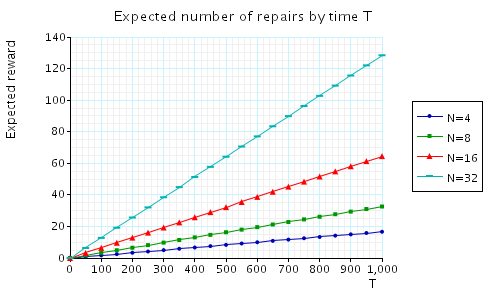# Workstation Cluster

(Haverkort, Hermanns & Katoen)

### Introduction

This case study is based on a cluster of workstations, taken from [HHK00]. The system comprises two sub-clusters with N workstations in each, connected in a star topology. The switches connecting each sub-cluster are joined by a central backbone. All components can break down and there is a single repair unit to service all components.We define two levels of Quality of Service (QoS):

• minimum QoS: at least 3N/4 workstations are operational and connected via switches and backbone;
• premium QoS: at least N workstations are operational and connected via switches and backbone.

The model is represented as a CTMC. The PRISM source code is given below.

```// Workstation cluster [HHK00]
// dxp/gxn 11/01/00

ctmc

const int N; // Number of workstations in each cluster
const int left_mx = N; // Number of work stations in left cluster
const int right_mx = N; // Number of work stations in right cluster

// Minimum QoS requires 3/4 connected workstations operational
const int k = floor(0.75*N);
formula minimum = (left_n>=k & toleft_n) |
(right_n>=k & toright_n) |
((left_n+right_n)>=k & toleft_n & line_n & toright_n);

// Failure rates
const double ws_fail = 1/500; // Single workstation: average time to fail = 500 hrs
const double switch_fail = 1/4000; // Switch: average time to fail = 4000 hrs
const double line_fail = 1/5000; // Backbone: average time to fail = 5000 hrs

// Left cluster
module Left

left_n : [0..left_mx] init left_mx; // Number of workstations operational
left : bool; // Being repaired?

[startLeft] !left & (left_n<left_mx) -> 1 : (left'=true);
[repairLeft] left & (left_n<left_mx) -> 1 : (left'=false) & (left_n'=left_n+1);
[] (left_n>0) -> ws_fail*left_n : (left_n'=left_n-1);

endmodule

// Right cluster
module Right = Left[left_n=right_n,
left=right,
left_mx=right_mx,
startLeft=startRight,
repairLeft=repairRight ]
endmodule

// Repair unit
module Repairman

r : bool; // Repairing?

[startLeft]    !r -> 10 : (r'=true); // Inspect Left
[startRight]   !r -> 10 : (r'=true); // Inspect Right
[startToLeft]  !r -> 10 : (r'=true); // Inspect ToLeft
[startToRight] !r -> 10 : (r'=true); // Inspect ToRight
[startLine]    !r -> 10 : (r'=true); // Inspect Line

[repairLeft]    r -> 2     : (r'=false); // Repair Left
[repairRight]   r -> 2     : (r'=false); // Repair Right
[repairToLeft]  r -> 0.25  : (r'=false); // Repair ToLeft
[repairToRight] r -> 0.25  : (r'=false); // Repair ToRight
[repairLine]    r -> 0.125 : (r'=false); // Repair Line

endmodule

// Line/backbone
module Line

line :   bool; // Being repaired?
line_n : bool init true; // Working?

[startLine] !line & !line_n -> 1 : (line'=true);
[repairLine] line & !line_n -> 1 : (line'=false) & (line_n'=true);
[] line_n -> line_fail : (line_n'=false);

endmodule

// Left switch
module ToLeft = Line[line=toleft,
line_n=toleft_n,
line_fail=switch_fail,
startLine=startToLeft,
repairLine=repairToLeft ]
endmodule

// Right switch
module ToRight = Line[line=toright,
line_n=toright_n,
line_fail=switch_fail,
startLine=startToRight,
repairLine=repairToRight ]
endmodule

// Reward structures

// Percentage of operational workstations stations
rewards "percent_op"
true : 100*(left_n+right_n)/(2*N);
endrewards

// Time that the system is not delivering at least minimum QoS
rewards "time_not_min"
!minimum : 1;
endrewards

// Number of repairs
rewards "num_repairs"
[repairLeft]    true : 1;
[repairRight]   true : 1;
[repairToLeft]  true : 1;
[repairToRight] true : 1;
[repairLine]    true : 1;
endrewards
```

### Model Statistics

The table below shows statistics for each model we have built. The first section gives information about the CTMC representing the model: the number of states and the number of non zeros (transitions). The second part refers to the MTBDD which represents this CTMC: the total number of nodes, the number of leaves (terminal nodes), and the amount of memory needed to store it. The last column gives the amount of memory a sparse matrix would need to represent the same CTMC.

 N: Model: MTBDD: Sparse: States: NNZ: Nodes: Leaves: Kb: Kb: 2 276 1,120 331 9 6.46 16.4 4 820 3,616 535 11 10.5 51.9 8 2,772 12,832 968 15 18.9 183 16 10,132 48,160 1,899 23 37.1 683 32 38,676 186,400 3,906 39 76.3 2,637 64 151,060 733,216 8,225 71 160 10,362 128 597,012 2,908,192 17,481 134 341 41,076 256 2,373,652 1.15e+7 37,272 262 728 162,581 512 9,465,876 4.6e+7 79,399 518 1,550 649,990

### Model Construction Times

The table below gives the times taken to construct the models. This process involves first building a CTMC (represented as an MTBDD) from the system description (in our module based language), and then computing the reachable states using a BDD based fixpoint algorithm. The total time required and the number of fixpoint iterations are given below.

 N: Construction: Time (s): Iter.s: 2 0.14 9 4 0.15 13 8 0.20 21 16 0.05 37 32 0.12 69 64 1.65 133 128 6.20 261 256 23.43 517 512 100.96 1,029

### Steady-State Probability Computation

The table below gives the times taken to compute the steady-state probabilities using the JOR method. The number of iterations required and the time taken using the MTBDD, sparse and hybrid engines is as follows.

 N: Time per iteration: (s) Iterations: MTBDD Sparse Hybrid 2 0.028 0.00026 0.00027 39 4 0.052 0.00031 0.00063 64 8 0.21 0.00085 0.0022 82 16 0.78 0.0035 0.0072 141 32 2.81 0.014 0.025 260 64 9.38 0.14 0.16 540 128 32.6 0.39 0.49 1,276 256 - - 2.16 3,634 512 - - 8.99 30,476

The steady-state probabilities allows use to compute properties such as:

• S=? [ "premium" ]: the long-run probability that premium QoS will be delivered.
• S=? [ !"minimum" ]: the long-run probability that QoS is below minimum.

### Model Checking

We have model checked the following properties:

• "The system will always be able to offer premium QoS at some point in the future." The corresponding CSL formula is given by:

P>=1 [ true U "premium" ]

Model checking times:

 N: Prob1 precomputation: Time (s): Iterations: 2 0.063 18 4 0.075 26 8 0.073 42 16 0.192 74 32 0.360 138 64 1.27 266 128 3.30 522 256 12.1 1034 512 44.3 2058

Conclusion: the property holds in all states
• "The chance that the QoS drops below minimum quality within T time units." The corresponding CSL formula is given by

P=? [ true U<=T !"minimum" ]

In the graph below we have plotted these probabilities from a range of values of T and N.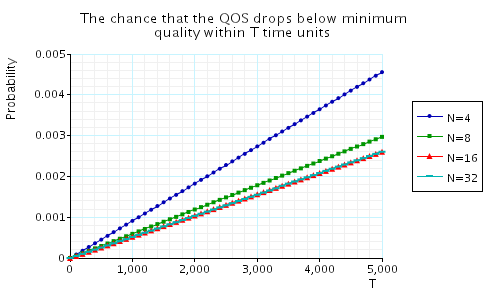• "When facing insufficient QoS, the (maximum) probability of facing the same problem after T time units." The corresponding CSL formula is given by

P=? [ true U[T,T] !"minimum" {!"minimum"}{max} ]

In the graph below we have plotted these probabilities from a range of values of T and N.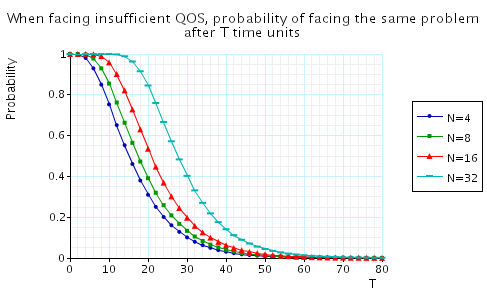• "The probability of going from minimum QoS to premium QoS within T time units." The corresponding CSL formula is given by

P=? [ true U<=T "premium" {"minimum"}{min} ]

In the graph below we have plotted these probabilities from a range of values of T and N.• "The probability of going from minimum QoS to premium QoS within T time units without violating the minimum QoS constraint along the way." The corresponding CSL formula is given by

P=? [ "minimum" U<=T "premium" {"minimum"}{min}]

In the graph below we have plotted these probabilities from a range of values of T and N.• "The probability that it takes more than T time units to recover from insufficient QoS." The corresponding CSL formula is given by

P=? [ !"minimum" U>=T "minimum" {!"minimum"}{max} ]

In the graph below we have plotted these probabilities from a range of values of T and N.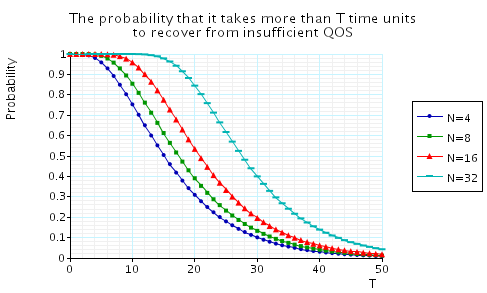• "The minimum percentage of operational workstations at time T when starting from below minimum QoS." The corresponding CSL formula is given by

R{"percent_op"}=?[ I=T {!"minimum"}{min} ]

In the graph below we have plotted these results from a range of values of T and N.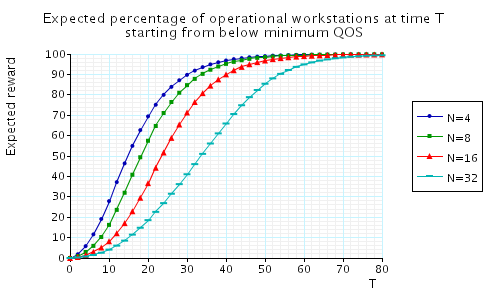• "The expected time that the system is below minimum QoS until time T." The corresponding CSL formula is given by

R{"time_not_min"}=?[ C<=T ]

In the graph below we have plotted these results from a range of values of T and N.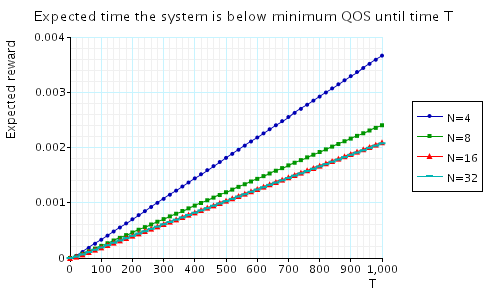• "The expected number of repairs by time T." The corresponding CSL formula is given by

R{"num_repairs"}=?[ C<=T ]

In the graph below we have plotted these results from a range of values of T and N.